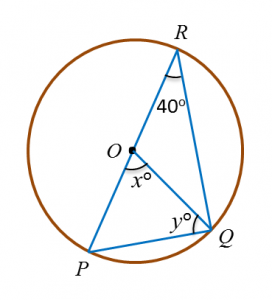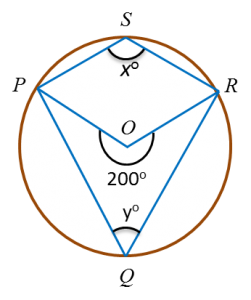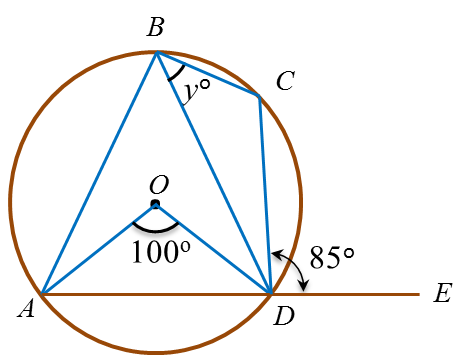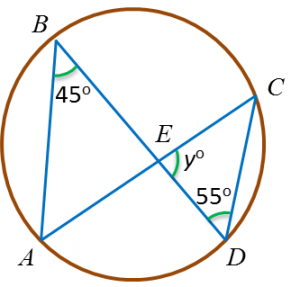# 4.2.2 Statistics (II), PT3 Focus Practice

4.2.2 Statistics (II), PT3 Focus Practice

Question 6:
Diagram below is a pie chart which shows the proportion of money allocated to five clubs.
The total amount of money allocated to Swimming Club and Squash Club is RM1800.
Calculate the total amount of money allocated to the five clubs.

Solution
:
Sum of the angles of the sectors for Swimming Club and Squash Club
= 360o – 65o – 85o – 90o
= 120o

Total amount of money allocated to the five clubs
$\begin{array}{l}=\frac{{360}^{o}}{{120}^{o}}×RM1800\\ =RM5400\end{array}$

Question 7:
Table below shows the number of students who watch four different TV channels in a week.

 TV Channels Number of Students News 8 Sports 15 Movies 25 Music 12
The information for sports channel is shown fully in the pie chart in the answer space.
Complete the pie chart to represent all the information in the Table.

:

Solution:
Total number of students
= 8 + 15 + 25 + 12 = 60
$\begin{array}{l}\text{News channel}\\ =\frac{8}{60}×{360}^{o}={48}^{o}\\ \\ \text{Movies channel}\\ =\frac{25}{60}×{360}^{o}={150}^{o}\\ \\ \text{Music channel}\\ =\frac{12}{60}×{360}^{o}={72}^{o}\end{array}$

Question 8:
Diagram below is a pie chart showing the number of mangosteens eaten by 4 boys.
Calculate
(a) the mean number of mangosteens eaten by a boy,
(b)   the angle of the sector representing Kenny.

Solution
:
(a)
$\begin{array}{l}\text{Mean}\\ =\frac{10+20+18+12}{4}\\ =\frac{60}{4}\\ =15\end{array}$

(b)
$\begin{array}{l}\text{Angle of the sector representing Kenny}\\ =\frac{18}{60}×{360}^{o}\\ ={108}^{o}\end{array}$

# 4.2.1 Statistics II, PT3 Focus Practice

4.2.1 Statistics II, PT3 Focus Practice

Question 1:
Given that the mode of the set of data 7, 5, 3, 2, 3, y, 7, 6 and 5 is 7, find the mean.

Solution:
If the mode is 7, then y = 7
$\begin{array}{l}\therefore \text{Mean}=\frac{7+5+3+2+3+7+7+6+5}{9}\\ \text{}=\frac{45}{9}\\ \text{}=5\end{array}$

Question 2:
The mean of the set of numbers 7, 2, x, 4, 5, 3, y is 5. The value of x + y is

Solution:
$\begin{array}{l}\text{Mean =}\frac{\text{sum of all values of data}}{\text{total number of data}}\\ \\ \frac{7+2+x+4+5+3+y}{7}=5\\ \therefore x+y+21=7×5\\ \text{}x+y=35-21\\ \text{}x+y=14\end{array}$

Question 3:
Table below shows the number of story books read by a group of pupils in a week.
 Number of books 1 2 3 4 5 Number of pupils 3 0 1 5 6
The median of the data is

Solution:
 Number of books 1 2 3 4 5 Number of pupils 3 0 1 5 6 Position 1 - 3 3 4 5 - 9 10 - 15
Number of pupils = 3 + 0 + 1 + 5 + 6 = 15
Middle position situated at 8th.
From the table, the position 8 has a value of 4, therefore the median of the data is 4.

Question 4:
Table below shows the scores for a group of pupils in a sport game.

 Score 1 2 3 4 5 Number of pupils 4 11 5 3 2
Calculate the percentage number of pupils who obtain scores more than the mode score.

Solution:
Mode score = 2
Number of pupils who obtain scores more than 2
= 5 + 3 + 2
= 10
Total number of pupils
= 4 + 11 + 5 + 3 + 2
= 25
Percentage number of pupils who obtain scores more than the mode score
$\begin{array}{l}=\frac{10}{25}×100%\\ =40%\end{array}$

Question 5:
The mean age of Alex, Michelle and their three children is 34 years. The mean age of their three children is 20 years.
Calculate the mean age of Alex and Michelle.

Solution:
Total age = 34 × 5 = 170 years
Total age of their three children
= 20 × 3
= 60 years
Total age of Alex and Michelle
= 170 – 60
= 110 years

Mean age of Alex and Michelle
$\begin{array}{l}=\frac{110}{2}\\ =55\text{years}\end{array}$

# 4.1 Statistics II

4.1 Statistics II

4.1.1 Pie Charts
1.   A pie chart is a graphic representation of data using sectors of a circle. The size of each sector shows the percentage of each category of data it represents.

Example:

2.   The percentage of data represented by each sector can be calculated by using the formula below.
$\text{Percentage of data}=\frac{\text{angle of sector}}{{360}^{o}}×100%$

3.   To construct a pie chart, the angle of each sector can be calculated by using the formulae below.
$\text{Angle of sector}=\frac{\text{data of each category}}{\text{overall data}}×{360}^{o}$

4.   Data can also be represented by pictograms, bar charts and line graphs.

5.   The choice of any these representations depends very much on the suitability of the data and the aim we wish to achieve in representing the data.

4.1.2 Mode, Median and Mean
1.   The mode of a set of data is the value of item which occurs most frequently.
Example:
3, 7, 6, 9, 7, 1, 5, 7, 2
Mode = 7

2.   When a set of data is given in a frequency table, the value or item which has the highest frequency is the mode.

3.   The median of a set of data is the value located in the middle of the set when the data is arranged in numerical order.
- If the total number of data is odd, then the median is the value in the middle of the set.
- If the total number of data is even, then the median is the average of the two middle values of the set

Example 1:
Find the medians of the following sets of data:
(a) 10, 9, 11, 6, 5, 8, 7
(b)   10, 9, 11, 6, 5, 8, 7,12

Solution:
(a) Number of data values = 7 ← (Odd number)
Rearranging the data in order of magnitude:
5, 6, 7, 8, 9, 10, 11
Therefore, median = 8
(b)   Number of data values = 8 ← (Even number)
Rearranging the data in order of magnitude:
5, 6, 7, 8, 9, 10, 11, 12

$\begin{array}{l}\therefore \text{Median}=\frac{8+9}{2}\\ \text{}=8.5\end{array}$

4.   When a set of data is given in a frequency table, the value situated in the middle position of the data is the median.

5.   The mean of a set of data is obtained by using formula below.

$\text{Mean =}\frac{\text{sum of all values of data}}{\text{total number of data}}$

Example:
Find the mean of the following sets of data items:
-5, -2, -1, 7, 4, 9
Solution:
$\begin{array}{l}\text{Mean}=\frac{\left(-5\right)+\left(-2\right)+\left(-1\right)+7+4+9}{6}\\ \text{}=\frac{12}{6}\\ \text{}=2\end{array}$

6.   When data is given in a frequency table, the mean can be found by using the formula below.

$\text{Mean =}\frac{\text{sum of}\left(\text{value}×\text{frequency}\right)}{\text{total frequency}}$

Example:
The table below shows the scores obtained by a group of players in a game.
 Score 1 2 3 4 5 Frequency 5 12 8 15 10
Find the mean of the scores.

Solution:
$\begin{array}{l}\text{Mean}\\ =\text{}\frac{\text{sum of}\left(\text{score}×\text{frequency}\right)}{\text{total frequency}}\\ =\frac{\left(1×5\right)+\left(2×12\right)+\left(3×8\right)+\left(4×15\right)+\left(5×10\right)}{5+12+8+15+10}\\ =\frac{163}{50}\\ =3.26\end{array}$

# 3.2.2 Circles II, PT3 Practice

3.2.2 Circles II, PT3 Practice

Question 6:
Diagram below shows a circle with centre O. POR is a straight line.Find the value of x and of y.

Solution:
$\begin{array}{l}x=40×2\\ \text{}=80\\ \\ y=\frac{180-80}{2}\\ \text{}=50\end{array}$

Question 7:
Diagram below shows a circle with centre O.Find the value of x.

Solution:
$\begin{array}{l}\angle QRO={38}^{o},\angle ORS={44}^{o}\\ \angle QRS={38}^{o}+{44}^{o}={82}^{o}\\ {x}^{o}={82}^{o}×2\\ {x}^{o}={164}^{o}\\ x=164\end{array}$

Question 8:
Diagram below shows a circle. QTS is the diameter of the circle and PTR is a straight line.Find the value of x.

Solution:
$\begin{array}{l}\angle RPS={90}^{o}-{42}^{o}={48}^{o}\\ {x}^{o}={48}^{o}+{37}^{o}={85}^{o}\end{array}$

Question 9:
Diagram below shows a circle with centre O. PQR is a straight line.Find the value of x and of y.

Solution:
Angle at Q = 180o – 70o = 110o
x2 = exterior angle at Q
= 180o – 110o
= 70o
x = 70

yo = reflex angle at O
yo = 360o – (70o × 2)
yo = 220o
y = 220

Question 10:
In diagram below, ABCD and DEFG are straight lines.Find the value of x and of y.

Solution:

# 3.2.1 Circles II, PT3 Practice

3.2.1 Circles II, PT3 Practice

Question 1:
(a) In Diagram below, KLMLPN and MPO are straight lines.

Find the value of x and of y.

(b) In the diagram below, O is the centre of the circle. Find the value of(i) x   (ii) y

Solution:
(a)
$\begin{array}{l}\angle LOM=\angle LNM={y}^{o}={44}^{o}\\ \therefore y=44\\ \angle NMO=\angle NLO={41}^{o}\\ {x}^{o}={180}^{o}-{80}^{o}-{41}^{o}\\ \text{}={59}^{o}\end{array}$

(b)(i)
2x = 200o
x = 100o

(b)(ii)
+ y = 180o
100o + y = 180o
y = 80o

Question 2:
(a) In Diagram below, AOC and BCD are straight lines with centre O.Find the value of y.

(b) In diagram below, ABCD is a circle with centre O and ADE is a straight line.Find the value of y.

Solution:
(a)
$\begin{array}{l}\angle AOB={45}^{o}×2={90}^{o}\\ {y}^{o}={180}^{o}-{90}^{o}={90}^{o}\end{array}$

(b)

$\begin{array}{l}\angle ABD=\frac{1}{2}×{100}^{o}={50}^{o}\\ \angle CDE=\angle ABC={85}^{o}\\ {y}^{o}+{50}^{o}={85}^{o}\\ \text{}{y}^{o}={35}^{o}\\ \text{}y=35\end{array}$

Question 3:
(a)  In diagram below, ABCD is a circle. AEC and BED are straight lines.Find the value of y.

(b) Diagram below shows a circle KLMN with centre O.Find the value of x.

Solution:
(a)
$\begin{array}{l}\angle ABE=\angle ACD={45}^{o}\\ {y}^{o}={180}^{o}-{45}^{o}-{55}^{o}\\ {y}^{o}={80}^{o}\\ y=80\end{array}$

(b)
$\begin{array}{l}\angle OML=\angle OLM={42}^{o}\\ \left({x}^{o}+{42}^{o}\right)+\angle KNM={180}^{o}\\ {x}^{o}+{42}^{o}+{110}^{o}={180}^{o}\\ {x}^{o}+{152}^{o}={180}^{o}\\ {x}^{o}={180}^{o}-{152}^{o}\\ {x}^{o}={28}^{o}\\ x=28\end{array}$

Question 5:
In diagram below, ABC and DEF are straight lines.Find the value of x and of y.

Solution:

# 3.1 Circles II

(B) Identify the Properties of Chords
(1) A radius that is perpendicular to a chord divides the chord into two equal parts.